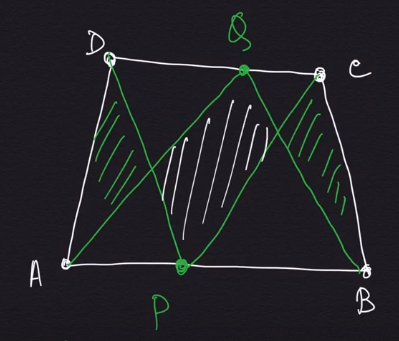How Cheenta works to ensure student success?
Explore the Back-Story

# Bose Olympiad Junior Level: ResourcesBose Olympiad Junior is suitable for kids in Grade 1, 2, 3 and 4.

## Curriculum

• Arithmetic
• Geometry
• Mathematical Puzzles

### Arithmetic

Basic skills of addition, subtraction and multiplication and division will be sufficient for attending arithmetic problems. Fundamental ideas about place-value system and ratios could be useful for Mains level.

Here is an example of an arithmetic problem that may appear in Bose Olympiad:

Suppose Ajit has 35 cheese sticks. Ajit makes Red Packs containing 3 sticks in each packet. Then Ajit makes Green packs containing 3 Red Packs each. Finally he makes Blue packs, each containing 3 Green Packs. How many unpacked sticks are there at the end of this process?

Key idea: Place Value System

### Geometry

A basic understanding is of shapes like triangle, circle, square is sufficient for prelims. Locus (path traced out by a moving point) is another key geometry topic that may appear. At the Mains level, the student may need notions of Area and Perimeter.

Here is an example of an geometry problem that may appear in Bose Olympiad:

Ayesha is running on a field such that his distances from two trees A and B are always equal. That is the distance of the position of Manoj from tree A is equal to the distance of the position of Manoj from tree B at any point of time. Then what is the shape of the path along which Ayesha is running?

Key idea: Locus

### Mathematical Puzzles

Mathematical puzzles may involve parallel channels, back tracking, greedy algorithm and recursive logic.

Here is an example of an puzzle problem that may appear in Bose Olympiad:

2019248 teams are playing in a knockout galactic football tournament. In this tournament no match ends in a draw and if you lose a match then you are out of the tournament. In the first round of the tournament the teams are paired up. In each subsequent round if even number of teams remain then they are again paired up, if odd number of teams remain then the highest scoring team is allowed to rest and directly go to the next round. How many matches are played in this tournament?

Key idea: one on one correspondence

## Reference Books

• Mathematics can be fun by Perelman
• Mathematical Circles for 3 to 8
• Lines and Curves by Vasiliyev
• Puzzles by Martin Gardner

Bose Olympiad Junior is suitable for kids in Grade 1, 2, 3 and 4.

## Curriculum

• Arithmetic
• Geometry
• Mathematical Puzzles

### Arithmetic

Basic skills of addition, subtraction and multiplication and division will be sufficient for attending arithmetic problems. Fundamental ideas about place-value system and ratios could be useful for Mains level.

Here is an example of an arithmetic problem that may appear in Bose Olympiad:

Suppose Ajit has 35 cheese sticks. Ajit makes Red Packs containing 3 sticks in each packet. Then Ajit makes Green packs containing 3 Red Packs each. Finally he makes Blue packs, each containing 3 Green Packs. How many unpacked sticks are there at the end of this process?

Key idea: Place Value System

### Geometry

A basic understanding is of shapes like triangle, circle, square is sufficient for prelims. Locus (path traced out by a moving point) is another key geometry topic that may appear. At the Mains level, the student may need notions of Area and Perimeter.

Here is an example of an geometry problem that may appear in Bose Olympiad:

Ayesha is running on a field such that his distances from two trees A and B are always equal. That is the distance of the position of Manoj from tree A is equal to the distance of the position of Manoj from tree B at any point of time. Then what is the shape of the path along which Ayesha is running?

Key idea: Locus

### Mathematical Puzzles

Mathematical puzzles may involve parallel channels, back tracking, greedy algorithm and recursive logic.

Here is an example of an puzzle problem that may appear in Bose Olympiad:

2019248 teams are playing in a knockout galactic football tournament. In this tournament no match ends in a draw and if you lose a match then you are out of the tournament. In the first round of the tournament the teams are paired up. In each subsequent round if even number of teams remain then they are again paired up, if odd number of teams remain then the highest scoring team is allowed to rest and directly go to the next round. How many matches are played in this tournament?

Key idea: one on one correspondence

## Reference Books

• Mathematics can be fun by Perelman
• Mathematical Circles for 3 to 8
• Lines and Curves by Vasiliyev
• Puzzles by Martin Gardner

This site uses Akismet to reduce spam. Learn how your comment data is processed.

1.Debjit Mullick says:

Sir ....Is there any such contest for UG or PG level students ...??

1.Dr. Ashani Dasgupta says:

Yes there will be. We will announce that soon.

### Knowledge Partner